Last Updated on 14 October, 2021 by Samuelsson

A moving average is a trading indicator that is employed in nearly all types of trading and is calculated by taking the average price of the x-last bars. The length of the lookback period can be altered to suit with the style of the investor and the market that is invested in. For example, to gauge the long term trend a setting of 200 might be appropriate, whereas detecting shorter term trend might require a much shorter length.

The moving average( MA) is probably the most well-known trading indicator there is. Long term investors swing traders, day traders and algo traders; they all use the moving average to guide them in their investments and trading decisions. The moving average, without doubt, is one of the most versatile and useful indicators there is.

In this article, we will learn what moving averages are, and how they can be used in trading and investing. We will also have a look at different common concepts that include moving averages, such as golden and death crosses.

Note that moving averages sometimes will be referred to as “MA”

## What Is A Moving Average?

A moving average is a technical indicator that is used to smoothen price action. This is done by calculating an average of the price, typically the close price, with the length of the lookback period. The average is then plotted on the chart, giving traders and investors the ability to visually determine the direction and strength of the trend.

The length of the moving average depends on what it’s used for. A longer lookback serves well to measure long term trends, while shorter lengths are better at discovering momentum and the short term direction of the market.  The most common and widely used setting for a moving average, are the 5, 10, 20, 50, 100,  and 200 bar moving averages.  Of these, the  200-day moving average is the most widely used indicator. Investors and traders often use it to gauge whether the market is bearish or bullish and to time the longer swings of the equity markets.  (This will be covered more in depth later in the article!)

### Which Is The Best Period Length?

Since moving averages can have different lookback lengths, you’re left with many choices as to what settings will work the best in your market and with your strategy. As always, there isn’t any definite answer as to what works best. Normally, optimal lengths vary with time, market, and strategy.

One of the strengths of moving averages lies in that other traders and investors use them in their decision making. If enough people use, let’ say a 200- day moving average, that very moving average will leave its mark on the market. It becomes a sort of self-fulfilling prophecy. Once price approaches the commonly agreed resistance or support level, market participant’s eagerness to fuel price movement farther will decrease. Therefore, for those new to investments and trading, the standard average lengths are the best ones to start with.

## Different Types of Moving Averages

There are many moving averages to choose from, and at first sight, it might seem overwhelming. However, you will come very far just by using the first one on this list.  If you’re a beginner and find all the alternatives overwhelming, we recommend you, at least for now, not to care about the other more complicated versions.

### 1. Simple Moving Average (SMA)

The simple moving average is, exactly as it sounds, a simple, plain moving average.  Despite its simplicity, it’s one of the most used of all moving averages and provides solid guidance for investors and traders.  All data points that are part of the calculation are equally weighted, unlike, for example, the exponential moving average. That means that if you have a simple moving average with a length of 200 days, the price 200 days ago will have as large an impact on the calculation as the price one day ago.

#### How To Calculate the Simple Moving Average

To calculate the Simple Moving Average, you simply need to calculate the average of the data points, in this case closing prices, for the lookback length you’ve chosen. So, if you’re calculating the 200-day moving average, you will have to take the sum of all the 200 previous closes. and divide them by 200.

Simple moving average= (C1 + C2 + C3 + C4 + … + Cn) / n

where C= price

### 2. Exponential Moving Average (EMA)

The Exponential moving average is a more advanced version of the simple moving average and differs in that it doesn’t place the same weight on all data points. Instead, the most recent data points are more heavily weighted, while the further back in time the data points are, the less impact they have on the calculation.

For example, if calculating a 200-day exponential moving average, the close price 1 day ago will be the most significant of all data points, while the close price 200 days ago will be weighted less than all previous data points.  This makes the exponential moving average is a less lagging indicator than the simple moving average, which means that it responds faster to price action.

#### How To Calculate Exponential Moving Average

The calculation of the exponential moving average is somewhat more complicated than the one of the simple moving average. For traders and investors, knowing how the exponential moving average works in theory and practice is enough. However, for those who wish to understand the calculation in detail, it’s provided below:

To calculate the exponential moving average you need to:

1. Calculate the Simple moving average
2. Calculate the weighting factor
3. Calculate the exponential moving average

We already know how to calculate the simple moving average. However, to calculate the weighting factor, we need a new formula:

2 ÷ (time period + 1)

So, if we have a 200-day Exponential moving average, the calculation becomes:

2 ÷ (200+ 1) =0.01

In this case, our weighting factor is 0.01. This means that the most recent close is given a weight of 1%.

The last step is to put the weighting factor, the simple moving average, into the following formula:

Exponential moving average=  (Close – EMA(previous period)} x weighting factor + EMA(previous period))

### 3. Volume Weighted Average Price (VWAP)

The volume-weighted Average Price, is a moving average that’s not only calculated on price but also volume. Higher volume means that the price is of higher significance. Therefore, the WVAP is calculated to place more weight on high volume bars, and vice versa.

One core difference of the VWAP to other moving averages is that it’s reset every day.

#### How to Calculate Volume Weighted Average Price

To calculate the VWAP, you take the sum of the volume multiplied by the price and divide it by the sum of the volume.

WVAP= (∑volume*Price)/∑Volume

### 4. Weighted Moving Average (WMA)

The Weighted moving average is another type moving average that places different weight on data points. In that regard, it’s reminiscent of the exponential moving average. However, while the weighting of the exponential moving average, of course, is of exponential nature, meaning that the rate of decrease is exponential, the decrease in the weighting of the weighted moving average is not. Instead, the weighting difference between one price and the preceding price is the same throughout the whole length of the moving average.

#### How To Calculate Weighted Moving Average

The weighted moving average is calculated by multiplying the price by its weighting factor and then calculating the sum of all data points. Here is the formula:

Weighted Moving Average = ( Price * x + Price(1) * x-1 + … Price( x-1 ) * 1) / ( x * ( x + 1 ) / 2 )

X= Time period

### 5. Hull Moving Average (HMA)

The Hull moving average was created by Alan Hull in 2005 and attempts to remove the lagging traits of moving averages while still being responsive, quick and smooth. For those who wish to know more about the HMA, we recommend you read more on Alan’s own website.

### 6. Triangular Moving Average(TMA)

The triangular moving average is a double smoothing moving average. This means that it first calculates an average for each data point, and then outputs the average of all those averages. Therefore, with the triangular moving average, you get a lot more lag than with other moving averages. Depending on your trading strategy or market, the increased lagginess could be to your detriment or advantage.

#### How to Calculate Triangular Moving Average

The calculation involves two steps.

1. First, calculate the simple moving average for each data point
2. Second, calculate the average of all data point averages

### 7. Fractal Adaptive Moving Average

The fractal adaptive moving average was invented by John Ehlers, and works in a rather unconventional way.

Instead of just taking the close price of each bar as most other moving averages do, it uses a custom measure that calculates the difference of the lowest lows and highest highs over different data points during the indicator length. This reading then goes through a couple of mathematical calculations before we arrive at the final FAMA value. The result is a moving average that dynamically adjusts its smoothing period, which in this case means a more responsive indicator.

### 8. Guppy Multiple Moving AverageGuppy Multiple Moving Average

Guppy Multiple Moving Average, or GMMA, outputs not only one indicator lines, but two whole clusters of moving averages.

The first cluster is made up of six short term averages, which usually are at  3, 5, 8, 10, 12, and 15 periods.

The second cluster consists of six long term moving average, and usually are set at 30, 35, 40, 45, 50, and 60 periods.

Since the Guppy Multiple Moving Average plots so many indicator lines, it becomes really easy to spot the main direction of the trend, with the distance between the lines showing the current trend strength.

_____________________________

### 9. McGinley Dynamic

The McGinley Dynamic indicator is a moving average that accounts for shifts in the market and the speed of the momentum, by adjusting the smoothing factor dynamically. As with some of the other indicators on this list, it’s done with the intention of creating a more dynamic indicator line.

_____________________________

### 10. Variable Index Dynamic Average (VIDYA)

The VIDYA moving average indicator may not be that common, and we understand if you haven’t yet heard of this indicator. The formula is fairly complex, but in short, it adjusts the smoothing factor to the current volatility levels of the market to achieve an indicator line that’s more relevant to the current market action.

________________________________

### 11. Moving Average Envelopes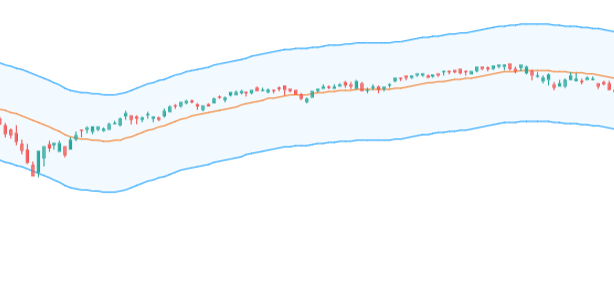Moving Average Envelope

A moving average envelop is an indicator that builds on the popular exponential moving average to create a price channel. This is done by plotting two lines, one above and another below the moving average, at a percentage distance from the moving average line itself.

The two bands are then used to measure things like breakouts and oversold and overbought conditions in the market.

## Simple Moving Average V.S Weighted Moving Averages

Both the simple moving average and the exponential moving average or other weighted moving averages have their place in a trader’s toolbox. One isn’t better than another and when switching between markets and strategies, you will find that they both work well in some settings, and worse in others.

However, the calculation of an exponential moving average makes it quicker to adapt to changes. This could have the benefit that it manages to quickly identifies new trends to profit from. On the other hand, that very same trait of the exponential moving average could make the trader get whipsawed in indecisive market conditions.

## What Can Moving Averages Be Used For?

Moving averages can be used for a variety of different purposes. They can identify the major trend and the momentum of the market, as well as provide indications of imminent bear or bull markets.

### Trends

When traders use moving averages to determine the direction of the trend, they do so by either looking at if the market is falling, or if it’s trading above the average. Below is an example of a falling moving average: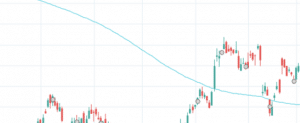Moving Average

While the moving average was falling, the market traded above it. Therefore, some traders would suggest that the trend isn’t falling, but rising.

#### Longer Lookback Periods

When detecting trends, the most common moving average is the 200-day moving average. Some traders also use shorter lookback lengths. However, if one wishes to detect the long term trend of markets, it’s important to choose a long lookback setting, and preferably, apply it to daily or even weekly bars.

Generally, the longer time a trend has persisted, the more certain we can be that it will continue into the future. That’s also one of the reasons why we expect equities to continue to rise in price.

### Momentum

The best way of determining the momentum of a security is with a shorter average length, and perhaps an exponential moving average. That’s because momentum tends to build up more quickly and not persist throughout time in the same manner as long term trends.

Generally, a lookback period of 20 or less is suitable to gauge short term momentum. Settings between 20-100, instead lend themselves better to detect medium-term momentum.

Regardless of what type of momentum traders or investors want to detect, it’s nearly always more practical to use more than one moving average. By plotting three average with different lookback periods, it’s possible to see how they diverge from each other. In that way, you may gauge short term momentum relative to medium-term momentum, and medium-term momentum relative to long term momentum.

Below you see how momentum accelerates, measured by the 5-, 10-, and 20-period moving average.Triple MA crossover

### Support And Resistance

When you look at charts with MA:s applied to them, you will find that moving averages with common settings, such as the 50, 100 or 200-period moving average, often act as support or resistance levels. In some markets, such as equities, this is of great concern for analysts, who often use the 200-day moving average as a regime filter.

Moving averages are also great to use as support and resistance levels. A support level is a level that price finds difficulties to fall under, and a resistance level is a level that price struggles to get above . See the image below for examples: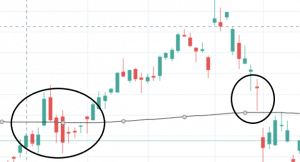Support and Resistance

As you can see, the price doesn’t turn around right on the moving averages. However, moving averages can still remain support or resistance, even if they’re penetrated. All types of support and resistance levels are approximative and should be used together with other indicators, patterns or edges to form a trading strategy.

#### Dynamic Support And Resistance Levels

When using moving averages as support and resistance, the levels become dynamic, and constantly change with price action. While typical support and resistance do that too, the moving average is unique in that it takes into account all the data points within the lookback period. With typical supports and resistance levels, it’s only one data point, such as the highest or lowest close, that determines where the resistance or support is.

### Stop Losses

The stop loss is critical for risk management and position sizing,  All trades, except for a few mean reversion type trades, should have a stop loss implemented. Most traders use a percentage or dollar-based stop loss, where the amount at risk is no more than, at most, a few percents of the account. However, there are also dynamic stop losses that adapt to market conditions and that adjust with the price movements of the market. These types of stop losses are called dynamic stop losses.

Dynamic stop losses are great when trying to catch long trends that persist for a long time. The stop loss level is moved with the advancing market, and triggered once there is a reversal of the trend.  In the image below you see an example of how this type of stop-loss works: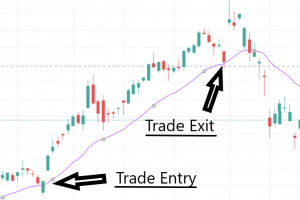moving average stop loss

### Profit Targets

Moving averages can also be used as a form of profit target. A profit target is a form of trade exit and works by exiting the trade once a certain amount of profit has been reached. Typically, traders use percentage or dollar-based profit targets.

By using a moving average as a profit target, the profit target level adapts to what the market does. In a rising market, the profit that is required for the trade to be exited is increased, and in a falling, provided that we’re going long, the opposite happens.

This could be great in situations where we want an exit mechanism that exits trades once the market has become oversold or overbought. Then, the price extending towards the average is an indication of that, regardless of where the price is in relation to the entry.moving average profit target

In the example above you see how we entered a trade, the market fell, and we exited the market once the market became oversold, despite incurring a loss.

### Market Regime Filters

Since moving averages can be used to determine whether the market is in an uptrend or downtrend, it can be used as a regime filter. Many markets behave differently in a bullish environment than in a bearish environment. Therefore, a moving average could help to restrict entries to only those market conditions where the strategy works the best.

In trading, one of the most common market regime filters is the 200-day moving average. Many trading strategies that go long in the equity markets work best when the market is rising. That is why it’s common to require the price to be over it’s 200-day moving average to enter a trade.

Some traders instead prefer to apply a 12-period moving average to monthly data. If the monthly close is above the 12-period moving average they assume that the market is bullish. Conversely, if the month closes below the 12-period moving average, they assume that the market is bearish. Applying a moving average to monthly data decreases the chances of getting whipsawed during times when the market moves indecisively. During those times, the low of the month may penetrate the 12-period moving average, but close above, thus still indicating a bullish market.

## Common Trading Signals Using  Moving Averages

Since moving averages are so common and useful, they’re part of many investing and trading strategies Two of the most common ones are the death cross and the golden cross.

### Moving Average Golden Crosses

Golden Crosses are when a shorter term moving average crosses over a longer-term moving average. It indicates that the short term trend has shifted and no longer is falling, like the long term trend. In effect, the golden cross becomes a moving average breakout, where the longer period moving average is the resistance level that’s breached.

Below is an example of a golden cross, using the 200 and 50-day moving averages: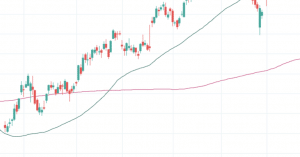MA Golden Cross

As you can see, both the 50 and 200-day moving averages first were falling. Then the 50-day average rose and crossed the now rising 200-day average. In other words, we had a golden cross.

The settings used in the example above, that is 50 and 200, are the standard ones that are most traders and investors use. However, as always, other moving average combinations could work as well. Just be sure not to optimize the lengths to force a golden cross into existence.  That’s just curve fitting!

#### False And True Golden Crosses

One common mistake many traders make, is to regard any moving average crossover as a golden cross. For there to be a true golden cross, the market must have come from a falling long term trend. In other words, the longer period moving average must be falling.

### Moving Average Death Crosses

Death crosses are the exact opposites of golden crosses. While a golden cross is an indication that a bullish market trend is imminent, the death cross warns about an approaching bear market.

The death cross is when a short period moving average crosses under a  longer period moving average.  As with the golden cross, the idea is that the reversal of the short term trend indicates the imminent turnaround of the market.Death cross

The most used moving average settings are the same as for the golden cross. That is 50 for the short period moving average, and 200 for the longer period moving average.

#### False And True Death Crosses

As with the golden cross, for the death cross to be true, the longer period moving average must be falling. If it’s not, that’s a false death cross.

### Moving Average Crossovers

A moving average crossover could be one of the following:

1.) the close crossing above or below the moving average

2.) A shorter-term moving average crossing above or below a longer-term moving average

The first type of crossover is the most basic one since it only requires one moving average. Since it’s the price that crosses over the moving average, it’s called a price crossover.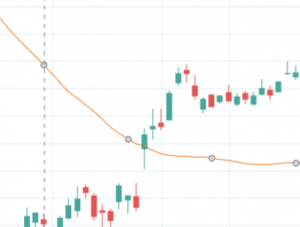Price crossover

The second type requires two moving averages. As you may have noticed, at a first glance, it seems no different from a death cross or a golden cross. However, for crossovers, we are not concerned with the moving average direction. The one and only criterion for a crossover are that one average crosses above another.  In the image below, we see several average crossovers: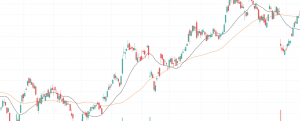Moving average crossovers

Many years ago, a strategy that went long on bullish crossovers, would have made a lot of money. However, with more and more efficient markets, that edge is now erased.

### Triple Crossover

By adding more moving averages to the chart, we can increase the validity of the signal. Most often traders use the five, 10-, and 20-day moving averages together, and wait for the five-day moving average to cross above the 10-day moving average(1). If that is followed by a crossover by the 10-day average over the 20-day average(2), the buy signal is issued.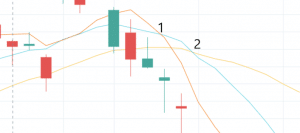Triple moving average crossover

The advantage of using several moving averages is that the number of false signals is reduced. Once the short term trend, defined as the 5-day average, has crossed over both averages, there is a greater likelihood that the trend will continue.

## Moving Average Based Indicators

Traders and investors don’t only use moving averages as a line on the chart. There are many trading indicators that use moving averages in their formula. Here we will give a basic introduction to the two most common average based indicators:

### Moving Average Convergence Divergence (MACD)

The MACD is one of the most popular indicators and is used to track the change in the relationship of two moving averages.  You calculate the relationship by subtracting the shorter period average from the long period average. The calculation, which is often made with the 12 and 26-period averages produces the MACD. Then you plot the MACD as a line and a histogram, together with a 9-day EMA of the MACD. This is the value that becomes the so-called signal line.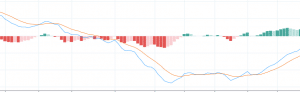MACD indicator

When using the MACD, traders often look for crossovers and divergences between price and the MACD line. Then, depending on whether the crossover or divergence takes place in positive or negative territory, traders give the signals varying significance.

### Bollinger Bands

Bollinger bands are two lines that you calculate by adding and subtracting the standard deviation to a moving average. You normally place the higher band two standard deviations above the moving average and the lower band two standard deviations under the average. Since the indicator incorporates standard deviation into the calculation, it’s always adapting to the current volatility level of the market.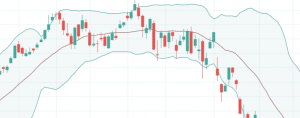Bollinger Bands

If the price moves towards one of the bands, it generally suggests that the security is becoming oversold or overbought.  The tightening and widening of the bands also give traders valuable information about the likelihood of imminent volatility increments. The longer time the bands have been compressed, the higher the chance that volatility is about to increase soon.

## Conclusion

Moving averages come in many forms and provide traders with a visual tool to determine the direction of the trend, as well as momentum. The different types of moving averages can be categorized into two groups; weighted and simple moving averages. The main difference is that weighted averages don’t place equal weight on all data points, whereas simple averages do.

Together with one more moving average, or only with price, there are many different entries that traders and investors can use. Golden crosses, death crosses and crossovers are some of them. There are also many indicators that have been developed from moving averages. Bollinger bands and MACD are two examples of such indicators.